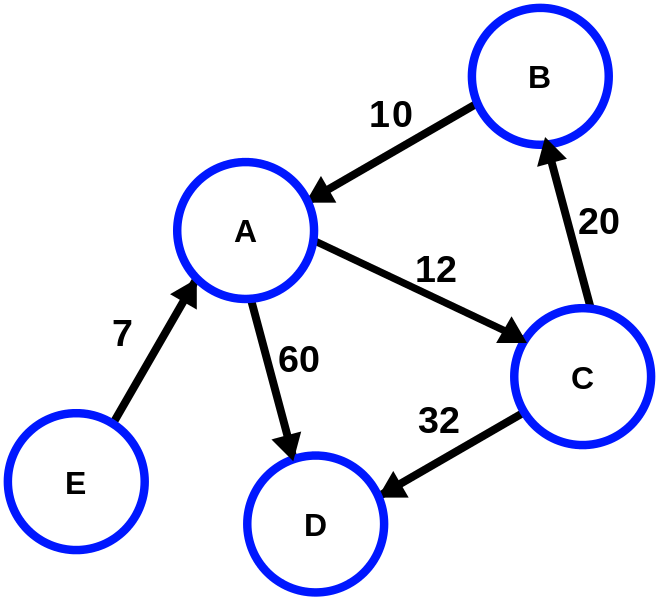# Weighted Edge Graph Data Structure in C++

So this data structure is one of the more complicated structures I’ll post about, I think I’ll be done with C++ for awhile.

A brief description of a graph, as explained by Wikipedia, “A graph data structure consists of a finite (and possibly mutable) set of vertices or nodes or points, together with a set of unordered pairs of these vertices for an undirected graph or a set of ordered pairs for a directed graph. These pairs are known as edges, arcs, or lines for an undirected graph and as arrows, directed edges, directed arcs, or directed lines for a directed graph. The vertices may be part of the graph structure, or may be external entities represented by integer indices or references.”

This being said, in simple, it is a structure with connected node points. An ideal use for it, is determining the shortest path. Take on the airplane analogy. What is the shortest path an airlines can put you through? There are so many ways to fly from one place to another, think of all the possible transfers, not just a direct flight. So a graph data structure would be perfect for storing each airport and the total distance to each one. Then when a customer comes to buy a ticket, it will be easy to calculate the shortest path for them to their destination.Just imagine A, B, C, D, E are different airports and the numbers show the distance to each

We are specifically going to look at graphs with weighted edges, from our analogy above, the weighted edge describes the distance to each airport. The weight determines which route the algorithm will take, ideally the lesser weight because it will be the shortest path.

```#include <iostream>
#include <vector>
#include <assert.h>

using namespace std;

class graph{
public:
static const size_t MAX = 20;

graph(){
numVerticies = 0;
edges = new int*[MAX];
labels = new char*[MAX];
for(size_t i = 0; i < MAX; i++){
edges[i] = new int[MAX];
labels[i] = nullptr;
}
for (size_t i = 0; i < MAX; i++){
for(size_t j = 0; j < MAX; j++) {
edges[i][j] = -1;
}
}
}

~graph() {
delete[] labels;
delete[] edges;
}

void add_edge(int source, int target, int weight=0) {
assert(source >= 0 && source < numVerticies);
assert(target >= 0 && target < numVerticies);

edges[source][target] = weight;
}

assert(numVerticies < MAX);
for(size_t i = 0; i < numVerticies + 1; i++) {
edges[i][numVerticies] = -1;
edges[numVerticies][i] = -1;
}
labels[numVerticies] = new char[strlen(vertexLabel) + 1];
strcpy(labels[numVerticies], vertexLabel);
numVerticies++;
}

void shortestPath(int start) {
int* distance = new int[numVerticies];
bool* marked = new bool[numVerticies];
for(size_t i = 0; i < numVerticies; i++){
marked[i] = false;
}
vector<int> path;
reset(distance);
distance[start] = 0;

cout << "Starting Dijkstra’s Shortest-Distance Algorithm with vertex " << labels[start] << endl;

int min = start;
while(path.size() < numVerticies) {
for (int v = 0; v < numVerticies; v++) {
if (!marked[v]){
if(distance[v] < distance[min]) {
min = v;
}
}
}
//Print it out

if (path.size() > 0){
int src = min;
int src_index = path.size() - 1;
while (edges[path[src_index]][min] != (distance[min] - distance[path[src_index]])){
src_index--;
}
src = path[src_index];
cout << "Vertex Pair " << labels[src] << "," << labels[min] << " ";
cout << "     Pair Cost: " << (distance[min] - distance[src]);
cout << "     Cost From Start: " << distance[min] << endl;
}

path.push_back(min);
marked[min] = true;

for (int i = 0; i < numVerticies; i++) {
if(is_edge(min, i) && (distance[i] > (distance[min] + edges[min][i]))) {
distance[i] = edges[min][i] + distance[min];
}
}
for (int x = 0; x < numVerticies; x++) {
if (!marked[x]){
min = x;
break;
}
}
}

delete[] marked;
delete[] distance;
}

void reset (int *&dist) {
for (int i = 0; i < numVerticies; i++) {
dist[i] = 999999999;
}
}

void DFS(int start) {
assert(start >= 0 && start < numVerticies);

bool* marked = new bool[numVerticies];
for(size_t i = 0; i < numVerticies; i++){
marked[i] = false;
}

cout << "Starting DFS with vertex " << labels[start] << endl;

DFS(start, marked);

cout << endl;

delete[] marked;
}

private:
int** edges;
char** labels;
int numVerticies;

bool is_edge(int source, int target) {
assert(source >= 0 && source < numVerticies);
assert(target >= 0 && target < numVerticies);

return edges[source][target] > -1;
}

void DFS(int start, bool marked[]){
assert(start >= 0 && start < numVerticies);

marked[start] = true;

cout << labels[start] << " ";

for (int i = 0; i < numVerticies; ++i) {
if(edges[start][i] > -1 && !marked[i]) {
DFS(i, marked);
}
}
}
};
```

Good luck and happy programming!

This site uses Akismet to reduce spam. Learn how your comment data is processed.